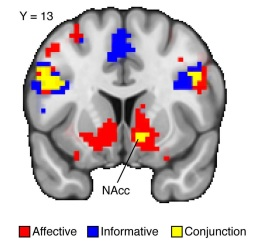# image display issue

Dear List experts,

I have a quick question. How can we overlay different results to the mask, using AFNI? I read a paper - the authors used this method, but with FSL (perhaps fslview tool). In that paper plot, there are two conditions displayed in the same task: affective display, informative display. They also showed the conjunction display with the same mask. The example display is attached. Does any one try this with AFNI? With AFNI, we only have one function “overlay” for one time, so it seems that it less likely. Any suggestions?

JHowdy-

This is a pretty common request, but, in short, AFNI giveth just one overlay.

You can join the data sets offline and then view the results. For example, if you have two masks of data in DSET1 and DSET2, you could make a new set where the value of voxels only in the DSET1 mask is 1, only in the DSET2 mask is 2, and both in DSET1 and DSET2 is 3:

``````
3dcalc -a DSET1 -b DSET2 -expr 'a+2b' -prefix COMBO_1and2

``````

… and you could make a colorbar of 3 colors of your heart’s desire.

How does that sound?

More complicated dsets (i.e., non-binary masks) can still be combined, as well, using logical operators to control what happens in overlapping situations.

–pt

Hi, PT,

1), why is the calculation expression is 'a+2b", rather than 'a+b"? Does it mean that your 'a+2b" stands for DSET1, DSET2, and both in DSET1 and DSET2? I assume that 'a+b" is equal to show the value of voxels from DSET1 and DSET2. Am I correct? How to obtain the both in DSET1 and DSET2, and get the image as what I show in the first post?

1. What is your method to make the colorbar?

Thank you very much,
j

Just to clarify and reiterate Paul’s explanation, a=dset1, b=dset2, the conjunction is set up in that formula to be 3. More specifically, these are the masks for dset1 and dset2 (affective, informative). If you set b=dset2 (say “informative” in your example), you could use just 1b, but that would give you only a value of 1, and that’s the same as as if dset1 is true at at that voxel, so you can’t tell the difference between 1 from affective and 1 from informative. By multiplying dset22, you can tell a difference because the informative mask gets a value of 2. The combination (conjunction) gets the sum of 3. The help for 3dcalc has a similar example, and there’s also another similar example in the afni11_ROI.pdf from the class handouts.

For the coloring, you could write and read in a palette file, but that may be a little trickier than what you want or need. First take a look at the paned colorbars, where the “**” appears below the color bar. Each of the colors in the color bar there is selectable to change the color or to move the range. Select one with 3 colors. Another way is to use one of the ROI color bars and set the range to the number of colors in that color bar, ROI_32 → range=32. You can make this happen automatically when you view the file with “3drefit -cmap INT_CMAP my_conjunctionmask_dset”.

A quick addition: you can do the calculations inside the AFNI GUI using the InstaCalc plugin, which is available from the InstaCorr drop-down menu in the Define Overlay control panel.

Great - thank you all very much,
J

Again, Daniel,

Perhaps I am using an old version of AFNI.

The command does not work with my computer. “3dcalc -a DSET1 -b DSET2 -expr ‘a+2b’ -prefix COMBO_1and2”, the error alarm is below.
PARSER error 5: Expected an operator
A+2B
…#
** FATAL ERROR: illegal expression!

but it works if I change the -expr ‘a+2b’ to -expr 'step(a)+2step(b)'. Does it mean that there is no expression of ‘a+2b’ , but it should be written as 'step(a)+2step(b)? What is the reason reason?

J

You are missing a multiplication operator between the ‘2’ and ‘b’. Try:

``````
3dcalc -a DSET1 -b DSET2 -expr 'a+2*b' -prefix COMBO_1and2

``````

–pt

Hi, PT,

Thanks for your clarification. I just tried and compared the two expressions below. It seems to me that the outputs are the same. Please let me know if I am wrong.

-expr ‘a+2b’
-expr 'step(a)+2
step(b)’ \

J

The expressions are not the same. The function “step()” is defined in the 3dcalc help as:

``````
step(x)    = {1 if x>0           , 0 if x<=0},

``````

… that is, wherever a dataset has a value greater than zero, step() of that value is 1, and where it ain’t, then step() of that value is 0.

If your ‘a’ and ‘b’ dsets are already binary masks, then “step(a)” would be equivalent to “a”, etc. However, you should be aware of the general functioning of the function.

-pt